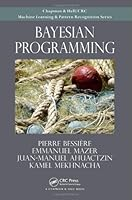# Bayesian Programming## Book Description

Probability as an Alternative to Boolean Logic

While logic is the mathematical foundation of rational reasoning and the fundamental principle of computing, it is restricted to problems where information is both complete and certain. However, many real-world problems, from financial investments to email filtering, are incomplete or uncertain in nature. Probability and Bayesian computing together provide an alternative to deal with incomplete and uncertain data.

Decision-Making Tools and Methods for Incomplete and Uncertain Data
Emphasizing probability as an alternative to Boolean logic, Bayesian Programming covers new methods to build probabilistic programs for real-world applications. Written by the team who designed and implemented an efficient probabilistic inference engine to interpret Bayesian programs, the book offers many examples that are also available on a supplementary website together with an interpreter that allows readers to experiment with this new approach to programming.

Principles and
Only requiring a basic foundation in , the first two parts of the book present a new methodology for building subjective probabilistic models. The authors introduce the principles of Bayesian programming and discuss good practices for probabilistic modeling. Numerous simple examples highlight the application of Bayesian modeling in different fields.

Formalism and Algorithms
The third part synthesizes existing work on Bayesian inference algorithms since an efficient Bayesian inference engine is needed to automate the probabilistic in Bayesian programs. Many bibliographic references are included for readers who would like more details on the formalism of Bayesian programming, the main probabilistic models, general purpose algorithms for Bayesian inference, and learning problems.

FAQs
Along with a glossary, the fourth part contains answers to frequently asked questions. The authors compare Bayesian programming and possibility theories, discuss the computational complexity of Bayesian inference, cover the irreducibility of incompleteness, and address the subjectivist versus objectivist epistemology of probability.

The First Steps toward a Bayesian Computer
A new modeling methodology, new inference algorithms, new programming languages, and new hardware are all needed to create a complete Bayesian computing framework. Focusing on the methodology and algorithms, this book describes the first steps toward reaching that goal. It encourages readers to explore emerging areas, such as bio-inspired computing, and develop new programming languages and hardware architectures.

Chapter 1: Introduction

Part I: Bayesian Programming Principles
Chapter 2: Basic Concepts
Chapter 3: Incompleteness and Uncertainty
Chapter 4: Description = Specification + Identification
Chapter 5: The Importance of Conditional Independence
Chapter 6: Bayesian Program = Description + Question

Part II: Bayesian Programming Cookbook
Chapter 7: Information Fusion
Chapter 8: Bayesian Programming with Coherence Variables
Chapter 9: Bayesian Programming Subroutines
Chapter 10: Bayesian Programming Conditional Statement
Chapter 11: Bayesian Programming Iteration

Part III: Bayesian Programming Formalism and Algorithms
Chapter 12: Bayesian Programming Formalism
Chapter 13: Bayesian Models Revisited
Chapter 14: Bayesian Inference Algorithms Revisited
Chapter 15: Bayesian Learning Revisited

Part IV: Frequently Asked Questions — Frequently Argued Matters
Chapter 16: Frequently Asked Questions and Frequently Argued Matters
Chapter 17: Glossary